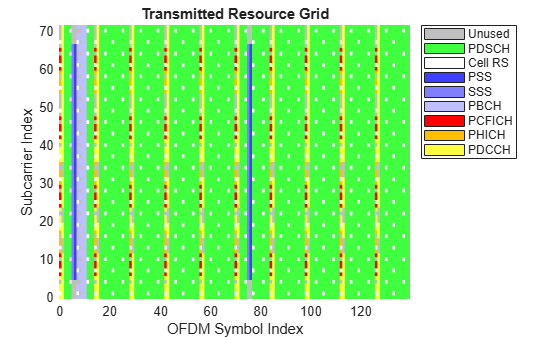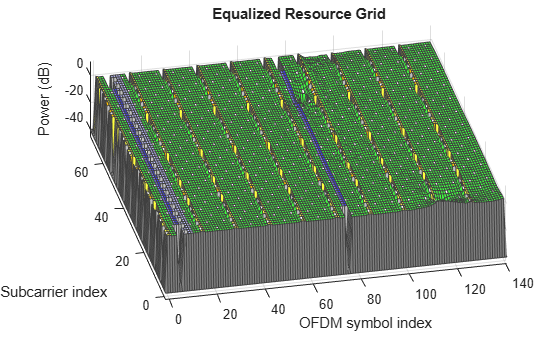This example shows how to implement an LTE transmit and receive chain, as shown in this figure.Generate an E-UTRA test model (E-TM) configuration. Use this configuration to generate the waveform and populate the resource grid.

```enb = lteTestModel('1.1','1.4MHz'); [txwave,txgrid,info] = lteTestModelTool(enb);```

Plot a graphical representation of the transmit resource grid.

```figure('Color','w'); helperPlotTransmitResourceGrid(enb,txgrid);```The figure shows a resource grid populated with E-TM 1.1 resource elements.

Simulate transmission through a fading channel propagation model.

```channel.ModelType = 'GMEDS'; channel.DelayProfile = 'EVA'; channel.DopplerFreq = 70; channel.MIMOCorrelation = 'Medium'; channel.NRxAnts = 1; channel.InitTime = 0; channel.InitPhase = 'Random'; channel.Seed = 17; channel.NormalizePathGains = 'On'; channel.NormalizeTxAnts = 'On'; channel.SamplingRate = info.SamplingRate; channel.NTerms = 16; rxwave = lteFadingChannel(channel,[txwave;zeros(25,1)]);```

Plot the time-varying power of the received waveform.

```figure('Color','w'); helperPlotReceiveWaveform(info,rxwave);```This plot shows the waveform power variation over time.

Perform frame synchronization.

```offset = lteDLFrameOffset(enb,rxwave); rxwave = rxwave(offset:end,:);```

Perform OFDM demodulation.

`rxgrid = lteOFDMDemodulate(enb,rxwave);`

Create a surface plot showing the power of the received grid for each subcarrier and OFDM symbol.

```figure('Color','w'); helperPlotReceiveResourceGrid(enb,rxgrid);```This plot shows the received grid power.

Estimate the channel and noise.

```cec.PilotAverage = 'UserDefined'; cec.FreqWindow = 9; cec.TimeWindow = 9; cec.InterpType = 'Cubic'; cec.InterpWindow = 'Centered'; cec.InterpWinSize = 3; [hest,nest] = lteDLChannelEstimate(enb,cec,rxgrid);```

Create a surface plot showing the magnitude of the channel estimate for each OFDM symbol across the subcarriers.

```figure('Color','w'); helperPlotChannelEstimate(hest);```This figure shows an estimate of channel magnitude frequency response.

Finally, perform minimum mean-square error (MMSE) equalization on the received grid.

`eqgrid = lteEqualizeMMSE(rxgrid,hest,nest);`

Create a surface plot of the power of the equalized resource grid, in dB.

```figure('Color','w'); helperPlotEqualizedResourceGrid(enb,eqgrid);```As can be seen the equalization flattened the power response across the resource grid.